# Physics Paper 2 Questions and Answers - KCSE Prediction Papers 2022

#### Instructions to Candidates

• Write your name and index number in the spaces provided above.
• Sign and write the date of examination in the spaces provided above. (c) This paper consists of two sections: A and B.
• Answer ALL the questions in sections A and B in the spaces provided. (e) ALL working MUST be shown clearly
• Mathematical tables and silent electronic calculators may be used.## Questions

SECTION A (25 MARKS)
Answer ALL the questions in this section in the spaces provided

1.
1. Distinguish between real and virtual Image (1mk)
2.  A pinhole camera forms an image of size 10cm. The object is 5m tall and 20m away from the pinhole. Find the length of the pinhole camera. (2mks)
2.
1. The figure 1 below shows a soft iron bar that’s placed in a coil near a free suspended magnet.State and explain the observation made when the switch is closed. (2mks)
2. Give a reason why attraction in magnetism is not regarded as a reliable method of testing for polarity. (1mk)
3. The figure 2 below shows an isolated negative charge placed closer to a negatively charged plate. Draw the electric field patterns. (1mk)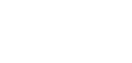4.
1. Define the term principal focus for a diverging lens. (1mk)
2. The figure 3 below illustrates an eye defect;1. Identify the defect. (1mk)
2. On the same diagram, sketch the appropriate lens to correct the defect and sketch rays to show the effect of the lens. (2mks)
5.
1. State the effect of pressure on the speed of sound in air. (1mk)
2. A boy stands 190m from a high wall and claps his hands. If he hears an echo1.3 Seconds later, calculate the speed of sound in air. (2mks)
6. State any two factors that determine the heating effect by an electric current. (2mks)
7. Figure 4 below shows an object, O placed 10 cm in front of a concave mirror whose radius of curvature, C is 40 cm.On the same figure, draw a ray diagram to show the position of the image formed. (3 mks)
8. Figure 5 shows the table of electromagnetic. Spectrum in the increasing order of wavelengths.
 P X-rays Q Infra-red
Identify the radiation marked P and Q (2mks)
9. State two reasons why the earth pin is normally longer than the other two pins in a three pin plug. (2mks)
10. The figure 6 below shows a cross section of a dry cell.1. Name the part labeled A (1 mark)
2. State the use of manganese (iv) oxide in the cell (1 mark)

SECTION B (55 marks)

1.
1.
1. In large currents, large resistors in parallel are preferred to low resistors in series. Explain (1mk)
2. State one condition under which ohm’s law is obeyed in a metal conductor. (1mk)
3.  A circuit constituting a battery, a metal wire, an ammeter and a switch connected in a series. The switch is closed and the ammeter reading noted. The metal wire is now heated. State observation on the ammeter reading and give a reason for your answer. (2mks)
2. In the figure 7 below, the voltmeter reads 2.4V when the switch is open. When the switch is closed, the voltmeter reads 2.1V and the ammeter reads 0.15A.
Determine the
1. E.m.f of the cell (1mk) b) Internal resistance of the cell (3mks)
2. Resistance of the bulb (2mks)
3. Explain why a voltmeter of high resistance is more accurate in measuring potential difference that one of low resistance (1mk)
4. Distinguish between electrical resistance and a resistor (1mk)
2.
1.
1. Define the term wavelength of a longitudinal wave (1mk)
2. The figure 8 below shows a displacement distance for a certain wave motion.
Determine1. The amplitude of the wave (1mk)
2. The wavelength of the wave (1mk)
3. Given that the frequency of the wave is 40Hz, determine the:
1. Periodic time (T) (1mk)
2. Speed of the wave (3mks)
2. Figure 9 below shows light rays from two coherent sources S1 and S2 falling on screen. Dark and bright fringes are observed between A and B1. State the function of S1 and S2 (1mk)
2. State how
1. Bright fringes are formed (1mk)
2. Dark fringes are formed (1mk)
3. Figure10 below shows plane water waves incident on a plane reflector placed at an angle to the path of the waves.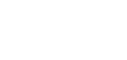Complete the diagram to show the reflected waves (2mks)
3.
1. State Snell’s law (1mk)
2. The Figure 11 below shows a ray of light travelling incident on air-kerosene interface.On the same diagram sketch the path of light as it traverses through the media showing the angle of refraction in air (3mks)
1. If the speed of light in kerosene is 2.08 x108 m/s, find the refractive index of kerosene. (speed of light in air=3.0 x108) (3mks)
2. Determine the angle of refraction in water (anw =4/3) (4mks)
4. The Figure 12 below shows a circuit with a battery, two switches, two capacitors and a volt meter1.  Determine
1. The charge on C1 when switch S1 is closed S2 open. (2mks)
2. The effective capacitance; CT when both switches S1 and S2 are closed. (3mks)
2. State and explain the expected final reading on the voltmeter when switch S1 is closed while S2 is open. (2mks)
3. Determine the expected reading on the voltmeter when switch S1 is closed for a while and opened and switch S2 is then closed. (2mks)
4. The Figure 13 below shows a polythene ball A and an aluminum coated polystyrene ball B.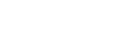Negative charges were introduced in each of the balls at the point marked x.
Draw similar balls and for each, indicate the final distribution of the charges .
(Use 6 dots to represent the charges in each case.) (2mks)
5.
1. The figure 14 below shows parts of a simple electric motor.Show the direction of the forces F acting on sides QR and PS of the coil. (1mk)
2. State the function of the devices A and B (2mks)
3. State two ways of increasing the rotation of the coil. (2mks)
4.
1. State one source of energy loss in a transformer (1mk)
2. How can the above energy loss be minimized. (1mk)
3. A transformer is used on the 240V A.C supply to deliver 9.0A at 80V to a heating coil. If 10% of the energy taken from the supply is dissipated in the transformer itself, what is the current in the primary winding? (3mks)## Marking Scheme

SECTION A (25 MARKS)
Answer ALL the questions in this section in the spaces provided

1.
1. Distinguish between real and virtual Image (1mk)
Real - formed by intersection of real rays while virtual formed by intersection of virtual rays.
2.  A pinhole camera forms an image of size 10cm. The object is 5m tall and 20m away from the pinhole. Find the length of the pinhole camera. (2mks)
V/U = hi/ho
V/2000 = 10/500
40cm or 0.4 m
2.
1. The figure 1 below shows a soft iron bar that’s placed in a coil near a free suspended magnet.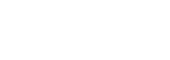State and explain the observation made when the switch is closed. (2mks)
Suspended magnet is repelled / moves away from the electromagnet
Reason: current flows forming a North pole at B
2. Give a reason why attraction in magnetism is not regarded as a reliable method of testing for polarity. (1mk)
Occurs either between unlike poles of magnet or between a magnet and magnetic materials.
3. The figure 2 below shows an isolated negative charge placed closer to a negatively charged plate. Draw the electric field patterns. (1mk)4.
1. Define the term principal focus for a diverging lens. (1mk)
Pt close to and parallel to the principal axis where all rays appear to diverge after refraction.
2. The figure 3 below illustrates an eye defect;1. Identify the defect. (1mk)
Myopia/ short sightedness
2. On the same diagram, sketch the appropriate lens to correct the defect and sketch rays to show the effect of the lens. (2mks)
5.
1. State the effect of pressure on the speed of sound in air. (1mk)
No effect.
2. A boy stands 190m from a high wall and claps his hands. If he hears an echo1.3 Seconds later, calculate the speed of sound in air. (2mks)
V= 2d/t
2 x 190/1.3
292.3m/s
6. State any two factors that determine the heating effect by an electric current. (2mks)
Resistance
Time of Heating
Current
7. Figure 4 below shows an object, O placed 10 cm in front of a concave mirror whose radius of curvature, C is 40 cm.On the same figure, draw a ray diagram to show the position of the image formed. (3 mks)
8. Figure 5 shows the table of electromagnetic. Spectrum in the increasing order of wavelengths.
 P X-rays Q Infra-red
Q. Visible light
9. State two reasons why the earth pin is normally longer than the other two pins in a three pin plug. (2mks)
Opening the socket blinds
Conducting any leaked charges.(earthing)
10. The figure 6 below shows a cross section of a dry cell.1. Name the part labeled A (1 mark)
Ammonium chloride paste
2. State the use of manganese (iv) oxide in the cell (1 mark)
Depolarizing agent/ depolarizer
or
oxidising hydrogen gas to water.

SECTION B(55 marks)

1.
1.
1. In large currents, large resistors in parallel are preferred to low resistors in series. Explain (1mk)
Parallel resistors- lower effective/ total resistance than those series
2. State one condition under which ohm’s law is obeyed in a metal conductor. (1mk)
Temperature
Pressure
Length
Cross-section area
3.  A circuit constituting a battery, a metal wire, an ammeter and a switch connected in a series. The switch is closed and the ammeter reading noted. The metal wire is now heated. State observation on the ammeter reading and give a reason for your answer. (2mks)
Resistance of a metal increases with increase in heat thue low current registered.
2. In the figure 7 below, the voltmeter reads 2.4V when the switch is open. When the switch is closed, the voltmeter reads 2.1V and the ammeter reads 0.15A.
Determine the
1. E.m.f of the cell (1mk)
2.4 V
2. Internal resistance of the cell (3mks)
Lost voltage = 2.4 -2.1 = 0.3V
Ir= 0.3
0.15r = 0.3
r= 2.0Ω
3. Resistance of the bulb (2mks)
V= IR
2.4= 0.15R
R=14Ω

Or
E=I(R+r)
2.4= 0.15(R+2)
R=14Ω
3. Explain why a voltmeter of high resistance is more accurate in measuring potential difference that one of low resistance (1mk)
Allows negligible/ No current to flow thus not interfering the current in the circuit.
4. Distinguish between electrical resistance and a resistor (1mk)
Resistance- opposition offered by a conductor to the flow of current resistors- a device designed to oppose current
2.
1.
1. Define the term wavelength of a longitudinal wave (1mk)
Distance between any two successive compressions or rarefaction
2. The figure 8 below shows a displacement distance for a certain wave motion.
Determine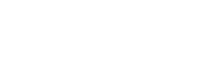1. The amplitude of the wave (1mk) ii) The wavelength of the wave (1mk)
10cm
2. Given that the frequency of the wave is 40Hz, determine the:
20cm
3.
1. Periodic time (T) (1mk)
T= i/f = 1/40 = 0.0255

2. Speed of the wave (3mks)
V= λf
0.2 x 40
8m/s
2. Figure 9 below shows light rays from two coherent sources S1 and S2 falling on screen. Dark and bright fringes are observed between A and B1. State the function of S1 and S2 (1mk)
Produce coherent waves that cause interference
2. State how
1. Bright fringes are formed (1mk)
Due to constructive interefrence/ or when a crest or trough meet
2. Dark fringes are formed (1mk)
Due to destructive interefrence or/ when a crest and trough of the two waves meet
3. Figure10 below shows plane water waves incident on a plane reflector placed at an angle to the path of the waves.Complete the diagram to show the reflected waves (2mks)3.
1. State Snell’s law (1mk)
Ratio of the sine of angle of incidence to the sine of angle of refrcation is constant for a given pair of the parallel

2. The Figure 11 below shows a ray of light travelling incident on air-kerosene interface.On the same diagram sketch the path of light as it traverses through the media showing the angle of refraction in air (3mks)
1. If the speed of light in kerosene is 2.08 x108 m/s, find the refractive index of kerosene. (speed of light in air=3.0 x108) (3mks)
η = c/v
3.0  x  108
2.08 x 108
1.442
2. Determine the angle of refraction in water (anw =4/3) (4mks)
aηk = sin 60/ sin r
1.442 = 0.8660/ Sin r
r= sIN-1(0.8660/1.442)
36.91º
kηw = Sin 36.91
Sinθw
But kηw  kηa .aηw
= 1/1.442  x 4/3 (0.9246)
θw =  Sin-1( Sin 36.91/0.9246)
= 40.50º
4. The Figure 12 below shows a circuit with a battery, two switches, two capacitors and a volt meter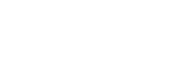1.  Determine
1. The charge on C1 when switch S1 is closed S2 open. (2mks)
Q=C1V
2 x 9
1.8 x 10-5C or 18MC
2. The effective capacitance; CT when both switches S1 and S2 are closed. (3mks)
C1 = C1 + C2
3 + 2
5μF or 5.0 x 10-6F
2. State and explain the expected final reading on the voltmeter when switch S1 is closed while S2 is open. (2mks)
9.0V
The p.d across a fully charged capacitor is equal to the voltage of the source.
3. Determine the expected reading on the voltmeter when switch S1 is closed for a while and opened and switch S2 is then closed. (2mks)
Charge on C1= 1.8 x 10-5C
S2- closed, 1.8 x 10-5 will be shared to charge C2
V=Q/C = 1.8  x  10-5 = 3.6V
5 x 10-6
4. The Figure 13 below shows a polythene ball A and an aluminum coated polystyrene ball B.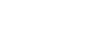Negative charges were introduced in each of the balls at the point marked x.
Draw similar balls and for each, indicate the final distribution of the charges .
(Use 6 dots to represent the charges in each case.) (2mks)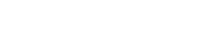5.
1. The figure 14 below shows parts of a simple electric motor.Show the direction of the forces F acting on sides QR and PS of the coil. (1mk)
2. State the function of the devices; (2mks)
1.    - Reverse direction of current every half cycle rotation
2.  - Conduct current/ lubricant
3. State two ways of increasing the rotation of the coil. (2mks)
Use a stronger magnet
Increase the no. of turns of wire
Increase current
Winding the wire on a soft iron core
4.
1. State one source of energy loss in a transformer (1mk)
Eddy currents
Hysterisis loss
Copper losses
2. How can the above energy loss be minimized. (1mk)
Follwo student's work above
(marks in d(ii) is tied to d(i))
3. A transformer is used on the 240V A.C supply to deliver 9.0A at 80V to a heating coil. If 10% of the energy taken from the supply is dissipated in the transformer itself, what is the current in the primary winding? (3mks)
Power output= VsIs
80 x 9= 720W
80 - 720
100 -?
Power input = 100 x 720 = 800
80

Power Input = VpIp
800 x 240 x Ip
Ip= 800/240
= 3.333A

• ✔ To read offline at any time.
• ✔ To Print at your convenience
• ✔ Share Easily with Friends / Students